# Complete the square calculatorThe problem of completing the square is to transform a second order degree polynomial as follows: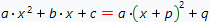where p and q are unknows parameters to be determined.

To determine unknown parameters p and q, transform the equality above as follows: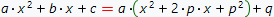and then, open the brackets: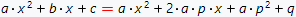To maintain the correct equality, equate the coefficients at the same degrees: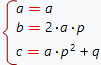The first equation of the system is the correct identity for any values of a, so it can be excluded. From the second equation, find the parameter p and substitute the resulting expression into the third equation: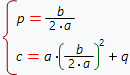Simplify third equation and find the value of the parameter q: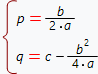Substitute values of parameters p and q into the first equation at the top of the page and get the formula for completing the square of second order degree polynomial: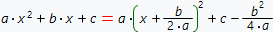The problem of completing the square often occurs when solving problems of rational functions integration. Moreover, by solving the problem of completing the square, one can obtain a formula for solving quadratic equations.

Our online calculator completes the square for second order degree polynomial with step by step solution.

Complete the square calculator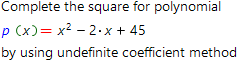Input second order polynomial: